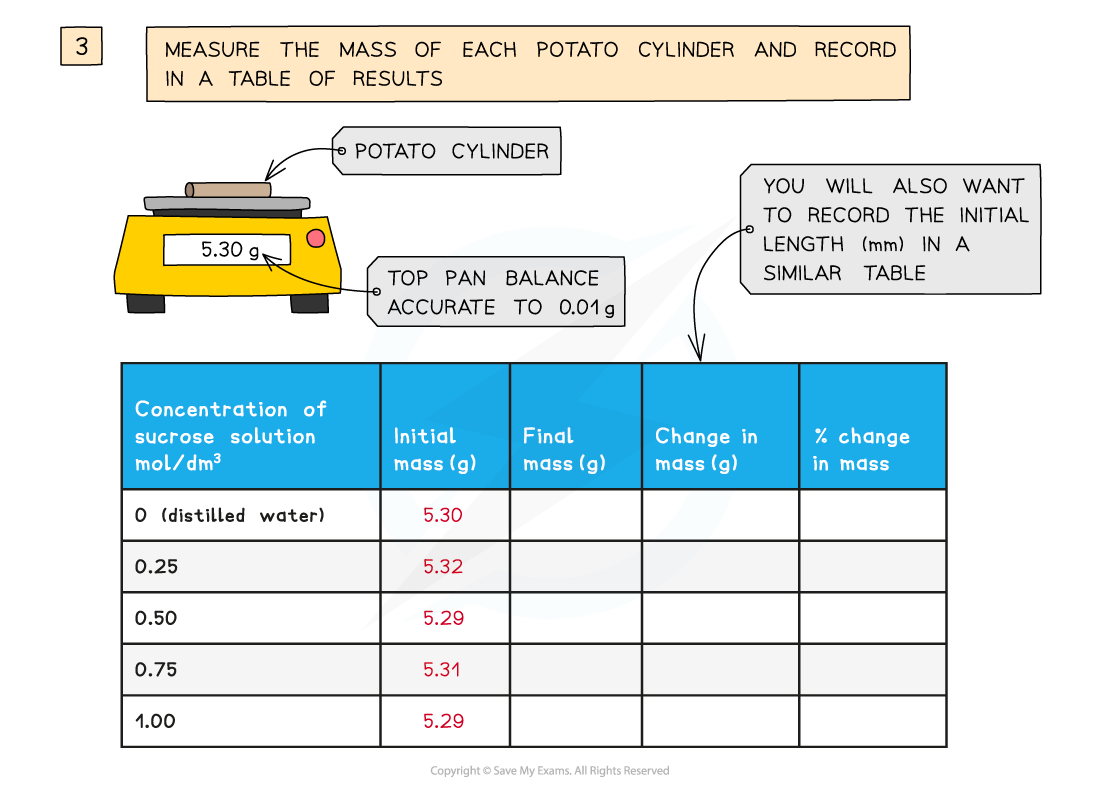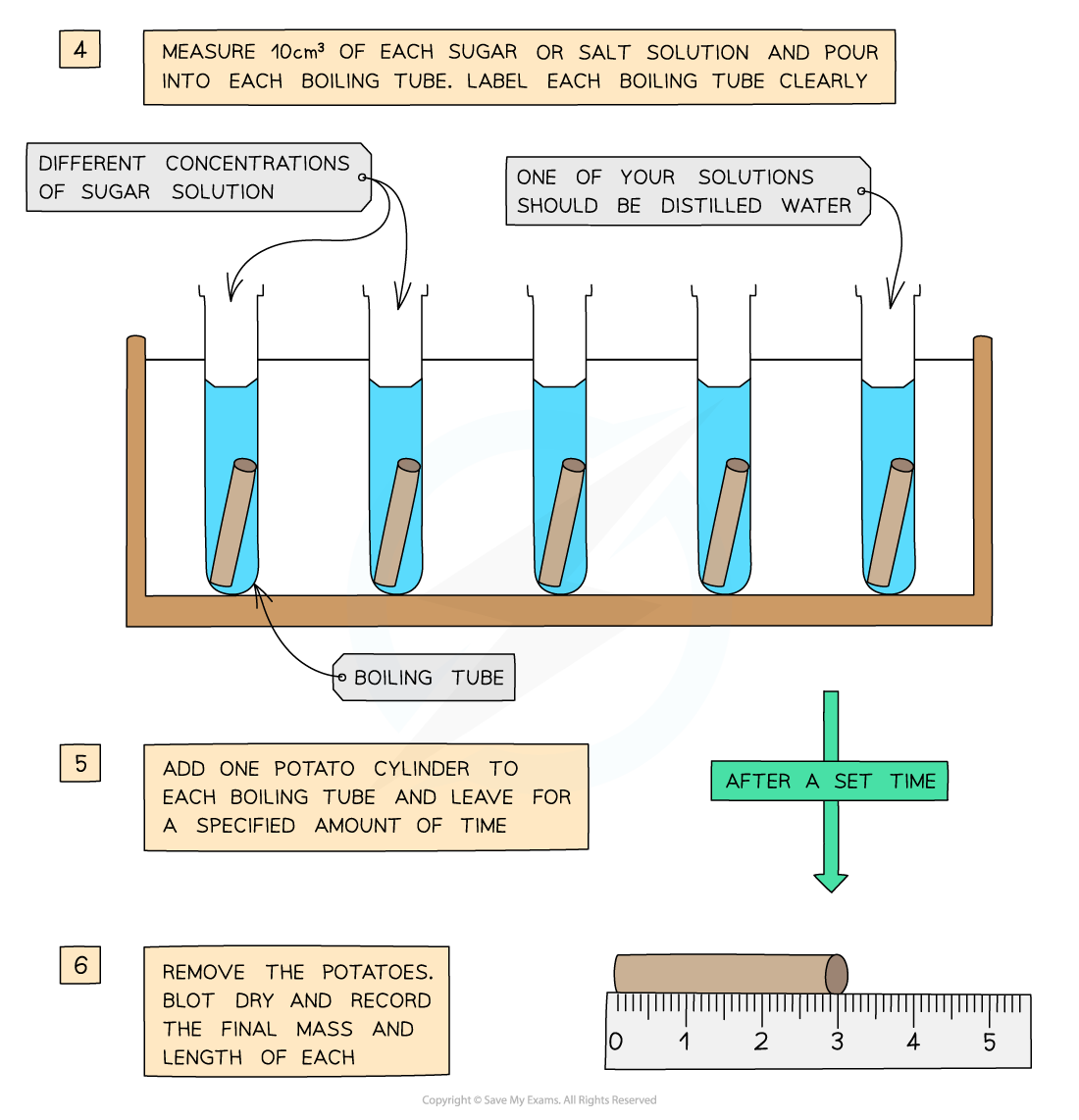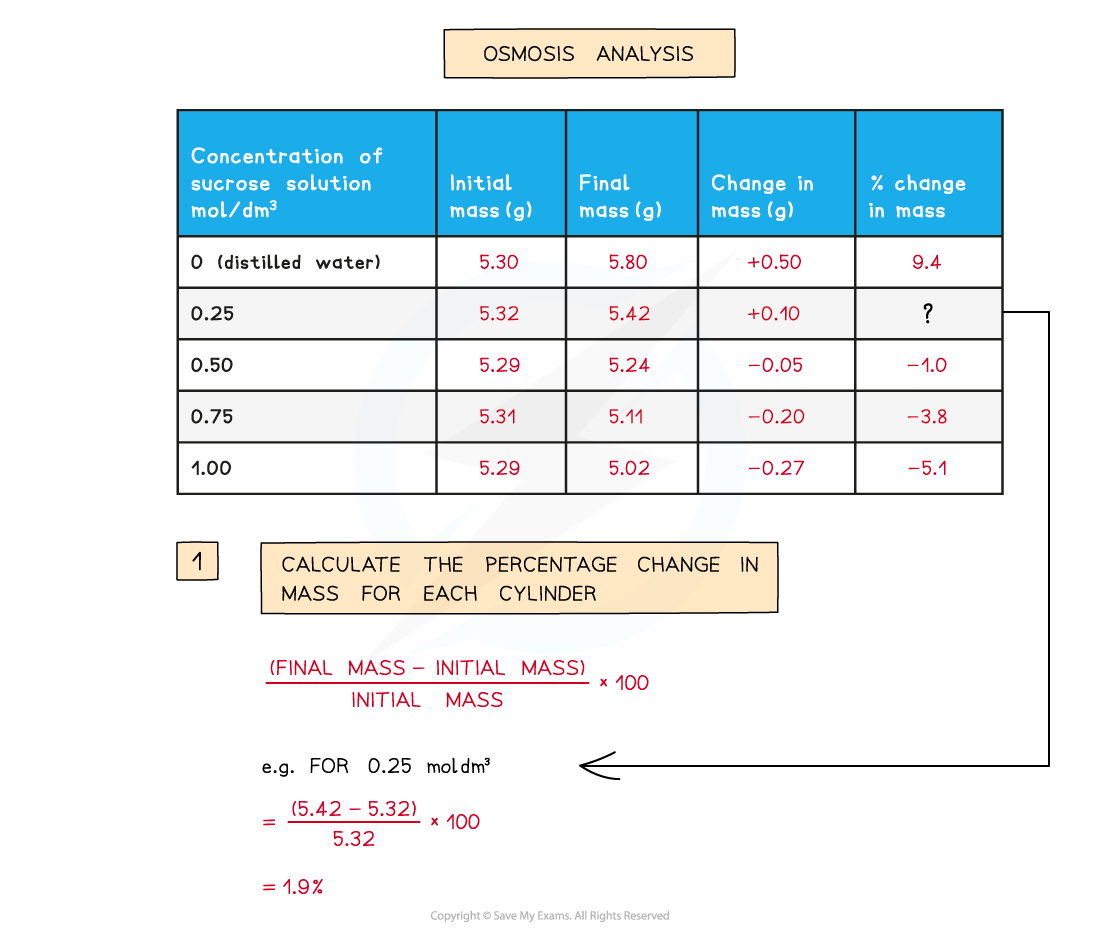# AQA A Level Biology复习笔记2.4.9 Water Potential Calibration Curves

### Required Practical: Investing Water Potential Using Calibration Curves

• It is possible to investigate the effects of immersing plant tissue in solutions of different water potentials and then use the results to estimate the water potential of the plant tissue itself
• The most common osmosis practical of this kind involves cutting cylinders of potato and placing them into solutions with a range of different water potentials (usually sucrose solutions of increasing concentration – at least 5 different concentrations are usually required)

#### Method

• The required number of potato cylinders are cut (one for each of the solutions you are testing – or more than one per solution if you require repeats)
• They are all cut to the same length and, once blotted dry to remove any excess moisture, their initial mass is measured and recorded before placing into the solutions
• They are left in the solutions for a set amount of time (eg. 30 minutes), usually in a water bath (set at around 30o)
• They are then removed and dried to remove excess liquid
• The final length and mass of each potato cylinder is then measured and recordedYou will need to use apparatus appropriately to measure out the volumes of your solutions and record your measurements

#### Analysis

• The percentage change in mass for each potato cylinder is calculatedTo find the percentage change in mass, the change in mass must be divided by the initial mass and then multiplied by 100

• A positive percentage change in mass indicates that the potato has gained water by osmosis (net movement of water from the solution into the potato) meaning the solution had a higher water potential than the potato
• This would make the potato cells turgid, as the water exerts turgor pressure (or hydrostatic pressure) on the cell walls – the potatoes will feel hard

• A negative percentage change suggests the opposite, that is, the solution had a lower water potential than the potato
• The potato cylinder in the strongest sucrose concentration will have decreased in mass the most as there is the greatest concentration gradient in this tube between the potato cells (higher water potential) and the sucrose solution (lower water potential)
• This means more water molecules will move out of the potato cells by osmosis, making them flaccid and decreasing the mass of the potato cylinder – the potato cylinders will feel floppy
• If looked at underneath the microscope, cells from this potato cylinder might be plasmolysed, meaning the cell membrane has pulled away from the cell wall

• If there is a potato cylinder that has neither increased nor decreased in mass, it means there was no overall net movement of water into or out of the potato cells
• This is because the solution that this particular potato cylinder was in had the same water potential as the solution found in the cytoplasm of the potato cells, so there was no concentration gradient and therefore no net movement of water into or out of the potato cells

### Maths Skill: Graph Intercepts

• The concentration of sucrose inside the potato cylinders can be found if a graph is drawn showing how the percentage change in mass changes with the concentration of sucrose solution – the point at which the line of best fit crosses the x-axis is the concentration of sucrose inside the potato cylindersA positive percentage change in mass indicates that the potato has gained water by osmosis (net movement of water from the solution into the potato) meaning the solution had a higher water potential than the potato. A negative percentage change suggests the opposite

### Maths Skill: Plotting Data

• Plotting data from investigations in the appropriate format allows you to more clearly see the relationship between two variables
• This makes the results of experiments much easier to interpret
• First, you need to consider what type of data you have:
• Qualitative data (non-numerical data e.g. blood group)
• Discrete data (numerical data that can only take certain values in a range e.g. shoe size)
• Continuous data (numerical data that can take any value in a range e.g. height or weight)

• For qualitative and discrete data, bar charts or pie charts are most suitable
• For continuous data, line graphs or scatter graphs are most suitable
• Scatter graphs are especially useful for showing how two variables are correlated (related to one another)

#### Tips for plotting data

• Whatever type of graph you use, remember the following:
• The data should be plotted with the independent variable on the x-axis and the dependent variable on the y-axis
• Plot data points accurately
• Use appropriate linear scales on axes
• Choose scales that enable all data points to be plotted within the graph area
• Label axes, with units included
• Make graphs that fill the space the exam paper gives you
• Draw a line of best fit. This may be straight or curved depending on the trend shown by the data. If the line of best fit is a curve make sure it is drawn smoothly. A line of best-fit should have a balance of data points above and below the line
• In some cases, the line or curve of best fit should be drawn through the origin (but only if the data and trend allow it)

#### Exam Tip

Questions involving osmosis experiments are common and you should be able to use your knowledge of osmosis to explain the results obtained. Don’t worry if it is an experiment you haven’t done – simply figure out where the higher concentration of water molecules is – this is the solution with the higher water potential – and explain which way the molecules move due to the differences in water potential.When drawing graphs, students often make mistakes when choosing the scales for their graphs. If possible, you should try to avoid scales that involve using parts of grid squares on the graph paper and instead try and use whole grid squares, as this makes it much easier to plot data points accurately.